# AP Statistics : How to find the median for a set of data

## Example Questions

### Example Question #1 : Univariate Data

Six homes are for sale and have the following dollar values in thousands of dollars:

535

155

305

720

315

214

What is the median value of the six homes?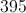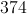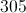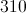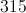Explanation:

The median is determined by ordering the values in the group from least to greatest: 155, 214, 305, 315, 535, 720.  The value directly in the middle is the median.  For instance, if there are five numbers, the third is the median.  Here, we have an even number of values so there is no value directly in the middle.  To find the median when there is no middle-most value, find the average of the two middle values.  The mean is determined by adding the two values and dividing by two (the number in the group):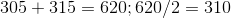### Example Question #1 : How To Find The Median For A Set Of Data

Find the median of the set.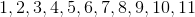Explanation:

The median is the middle value of the set in increasing order.

In this set of 11 entries, the median is the 6th entry of the set in increasing order, or 6.

### Example Question #3 : How To Find The Median For A Set Of Data

Find the median of the set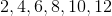Explanation:

The median is the middle value of the set in increasing order.

In this set of 6 (or any even number of) entries, the median is the mean of the two middle entries of the set in increasing order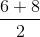or### Example Question #4 : How To Find The Median For A Set Of Data

Letbe a positive integer.

Find the median of the set.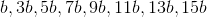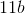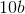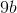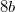Explanation:

The median is the middle value of the set in increasing order.

In this set of 8 (or any even number) entries, the median is the mean of the two middle entries of the set in increasing order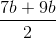or### Example Question #1 : How To Find The Median For A Set Of Data

Suppose a basketball team plays six games and scores the following points: 69, 78, 82, 69, 98, 85. Find the median.

82

69

80

75.5

80

Explanation:

To find the median of a sample with an even sample size, order the values from smallest to largest, take the two values in the middle, add them, and divide that by zero.

69, 78, 82, 69, 98, 85

69, 69, {78, 82} 85, 98

(78+82) / 2 = 80